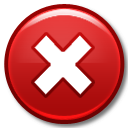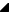﻿ TRSChartValues Properties# TRSChartValues PropertiesProperties

Name

DescriptionAbsSum

Returns the sum of the absolute values of the first dimension of the values in the chart. The   AbsSums property returns the absolute sum for any dimension in the chart (see   DimensionCount for more details).AbsSums[Integer]

Returns the summation of the absolute values for every dimension of the TRSChartValues in the chart. Use the     DimensionCount property to determine the number of dimensions in the chart value collection. This property is part of the metadata that the collection tracks about the chart values.

A chart value, depending on the chart type, can contain 1 or more dimensional values that define the chart value. For example, each chart value in a bar chart contains 1 value (defining the height of the bar), line chart values contains 2 dimensions: X and Y, and shape chart values contain 4 dimensions: X, Y, Width, and Height. See the Values and ValueCount properties of the TRSChartValue item for more information.DateTimeAxes

Represents property DateTimeAxes.DefaultDimension

Returns the     Values dimension that contains the default dimension or key dimension, i.e., the dimension that is most important to a chart. For 1D charts, like a pie chart, this value is 0. For 2D charts, this dimension is usually 1.

Use the     TimeDimension and the     DefaultDimension to find out the "important" dimensions of the TRSChartValue descendant.Note

The FMX.RS.ChartPanel.TRSChartValue.Value property uses this property to figure out which Values dimension to get or set.DimensionCount

Returns the number of dimensions each Value ( FMX.RS.ChartPanel.TRSChartValue ) will have in the chart.

A chart value, depending on the chart type, can contain 1 or more dimensional values that define the chart value. For example, each chart value in a bar chart contains 1 value (defining the height of the bar), line chart values contains 2 dimensions: X and Y, and shape chart values contain 4 dimensions: X, Y, Width, and Height. The number of dimensions in a chart is defined when the TRSChartValues collection is created. Every time a TRSChartValue item is created, its Values property will be initialized to the size of DimensionCount.DoNotNotify

Represents property DoNotNotify.HasCaptions

Specifies that at least one TRSChartValue in the collection has had a Caption assigned to it.IsDateTime

Controls whether the first (or only) dimension should be labeled as a date time or a regular floating point number. When this property is set to True, all the labels for this chart will be drawn as a Date + Time (controlled by the   DateTimeFormat of the axis).Items[Integer]

Lists the values in the chart. Use Items to access individual value in the collection. The value of the Index parameter corresponds to the Index property of FMX.RS.ChartPanel.TRSChartValue . It represents the position of the value in the collection.

The Items property is the default property for the TRSChartValues class. You can access this property with the abbreviation Values[index], which is equivalent to Values.Items[index].Limit

Limits the number of values (or FMX.RS.ChartPanel.TRSChartValue items) in the collection. It provides the chart the ability to limit its size automatically as values are added.

Once the Count property equals the Limit property, every time you Add another value, the 0-th value is deleted. This property makes it easy to ensure your chart values do not grow unbounded and creates an automatic sliding window display ofthe Limit number of points. Note that this is not necessarily the last Limit number of points added, as sorting the values (Sorted) can make which value is deleted unpredictable.

If you want an unlimited number of chart values, set Limit to RSChartConsts|NoLimit .MaxValue

Returns the maximum value of the first dimension of the values in the chart. The   MaxValues property returns the maximum value for any dimension in the chart (see   DimensionCount for more details).MaxValues[Integer]

Returns the maximum value for every dimension of the TRSChartValues in the chart. Use the     DimensionCount property to determine the number of dimensions in the chart value collection. This property is part of the metadata that the collection tracks about the chart values.

A chart value, depending on the chart type, can contain 1 or more dimensional values that define the chart value. For example, each chart value in a bar chart contains 1 value (defining the height of the bar), line chart values contains 2 dimensions: X and Y, and shape chart values contain 4 dimensions: X, Y, Width, and Height. See the Values and ValueCount properties of the TRSChartValue item for more information.MinValue

Returns the minimum value of the first dimension of the values in the chart. The   MinValues property returns the maximum value for any dimension in the chart (see   DimensionCount for more details).MinValues[Integer]

Returns the minimum value for every dimension of the TRSChartValues in the chart. Use the     DimensionCount property to determine the number of dimensions in the chart value collection. This property is part of the metadata that the collection tracks about the chart values.

A chart value, depending on the chart type, can contain 1 or more dimensional values that define the chart value. For example, each chart value in a bar chart contains 1 value (defining the height of the bar), line chart values contains 2 dimensions: X and Y, and shape chart values contain 4 dimensions: X, Y, Width, and Height. See the Values and ValueCount properties of the TRSChartValue item for more information.NeedMetaUpdate

Signals that metadata is out of date and needs to be recalculated by calling the CalculateMetaData methodNumDimensions

Specifies the number of dimensions the TRSChartValue's have. The number of dimensions specify the size of the   TRSChartValue.Values property and corresponds to all the floating point properties of a value (e.g., X, Y, Z, Width, Height, Size, etc).SelCount

Returns the number of   selected values in the collectionSortByDimension

Controls which dimension is used to sort the items in the collection (when     Sorted is True).

A chart value, depending on the chart type, can contain 1 or more dimensional values that define the chart value. For example, each chart value in a bar chart contains 1 value (defining the height of the bar), line chart values contains 2 dimensions: X and Y, and shape chart values contain 4 dimensions: X, Y, Width, and Height. The SortByDimension allows you to sort the items by the dimension (X usually but it could be Width in a shape chart, Level in a graph chart, etc). See the     Values and     ValueCount properties of the TRSChartValue item for more information.Sorted

Controls whether the values of the chart are sorted or not.

Set the Sorted property to True to force all values to be sorted in the collection based on the     SortByDimension and     SortOrder properties. When this property is False, the values are in the order that they were added or inserted into the collection (see Add or Insert methods).SortOrder

Controls whether the items are sorted in ascending or descending order. The FMX.RS.ChartPanel.TRSChartValues.SortByDimension property controls which values are used to do the sorting. The FMX.RS.ChartPanel.TRSChartValues.Sorted property controls whether the items will be sorted at all.Sum

Returns the summation of the first dimension of the values in the chart. The   Sums property returns the summation for any dimension in the chart (see   DimensionCount for more details).Sums[Integer]

Returns the summation of the values for every dimension of the TRSChartValues in the chart. Use the     DimensionCount property to determine the number of dimensions in the chart value collection. This property is part of the metadata that the collection tracks about the chart values.

A chart value, depending on the chart type, can contain 1 or more dimensional values that define the chart value. For example, each chart value in a bar chart contains 1 value (defining the height of the bar), line chart values contains 2 dimensions: X and Y, and shape chart values contain 4 dimensions: X, Y, Width, and Height. See the Values and ValueCount properties of the TRSChartValue item for more information.TimeDimension

Returns the     Values dimension that contains the time/x/horizontal dimension. For 1D charts, like a pie chart, this value is unassigned (DIMENSION_UNASSIGNED). For 2D charts, this dimension is usually 0.

Use the     TimeDimension and the     DefaultDimension to find out the "important" dimensions of the TRSChartValue descendant.VisibleCount

Returns the number of visible values in the collection (   Visible = True)

Top

RiverSoftAVG Charting Component Suite (RCCS) © 2005-2015, Thomas G. Grubb